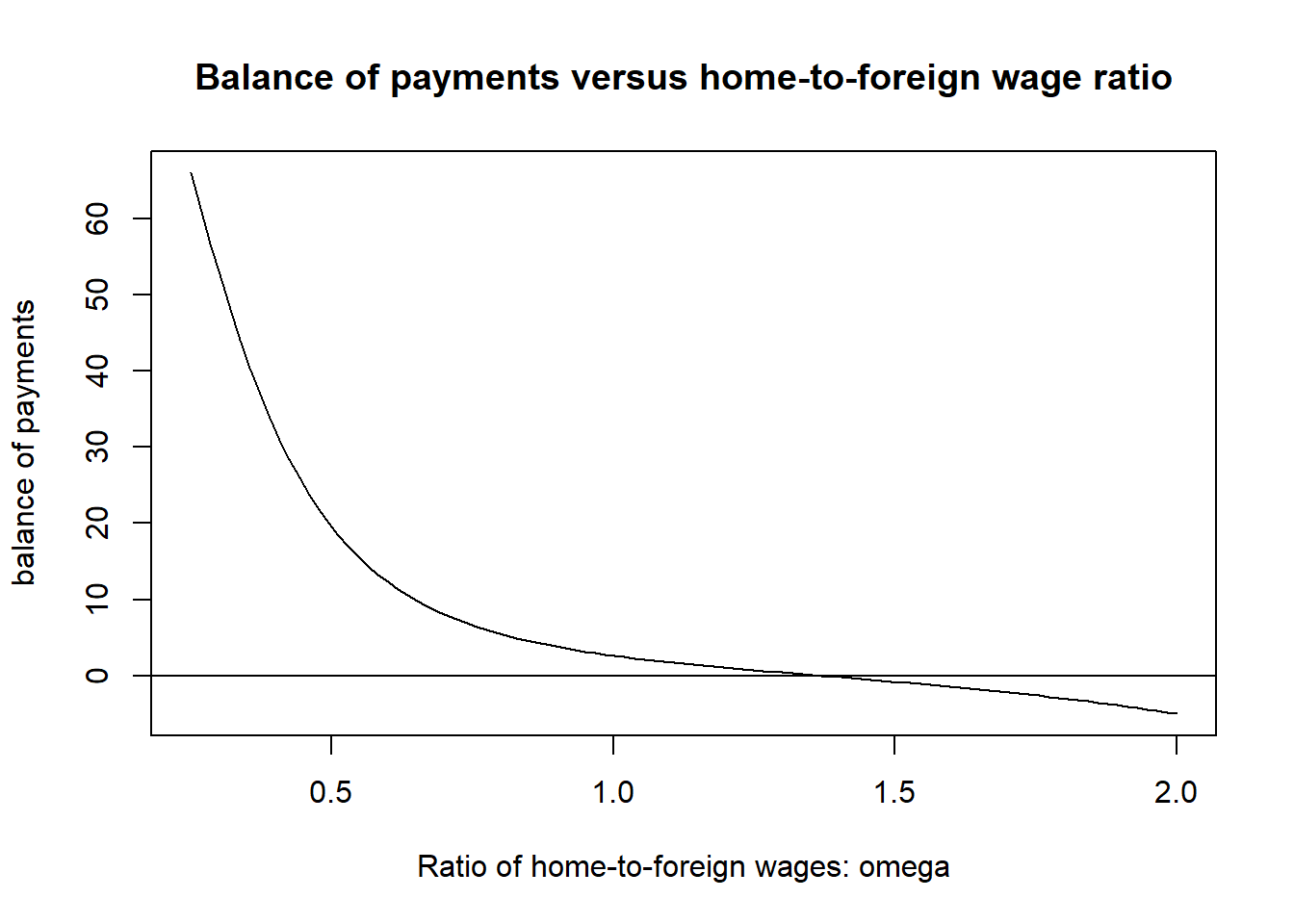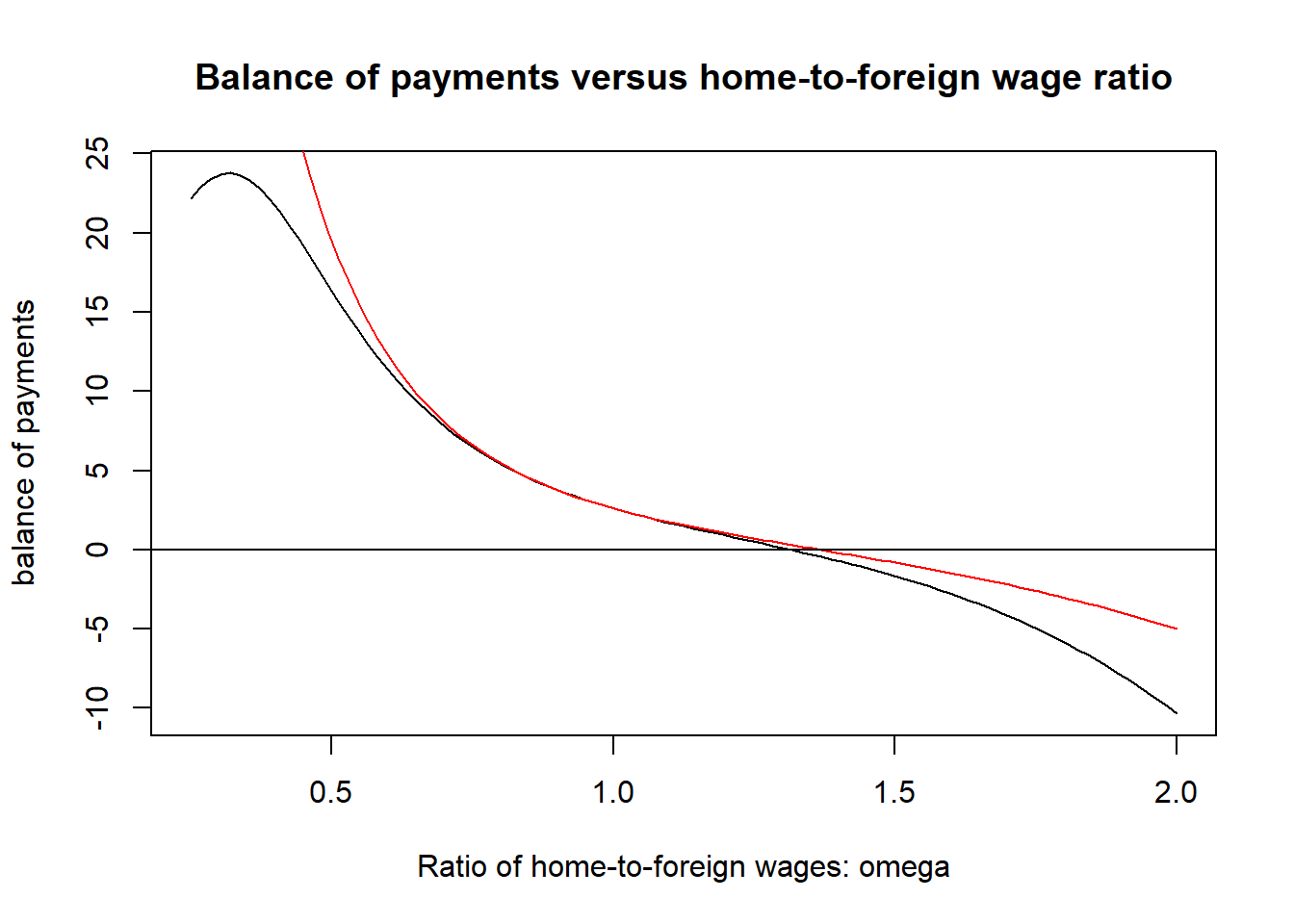In this notebook I simulate the model from Paul Krugman’s “Scale Economies, Product Differentiation, and the Pattern of Trade,” AER, 1980.

Main parameters:

$$\theta$$: from $$U=\sum_i c_i^{\theta}$$, where $$0<\theta<1$$

$$L,L^*$$: population of “home” and “foreign” (*) regions

$$\alpha,\beta$$: fixed and marginal cost parameters from $$l_i=\alpha+\beta*x_i$$

$$g$$: transportation cost, if $$1$$ unit is shipped only $$g$$ arrives in the other country

Wages and prices are not uniquely defined in autarky and in the case of trade only the ratio $$\omega=w/w^*$$ is defined. Therefore I normalize the wage of the home country to $$w=1$$, which will imply the price will be $$p=w*\beta/\theta=\beta/\theta$$ in equilibrium.

Lastly, in all equilibria the number of firms and output of each good is simply a function of the exogenous parameters:

$$x=\frac{\alpha\theta}{\beta(1-\theta)}$$, $$n=\frac{L(1-\theta)}{\alpha}$$ and $$n^*=\frac{L^*(1-\theta)}{\alpha}$$

#Main parameters:
theta=0.75
l.home=900
l.foreign=100
alpha=0.75
beta=0.5
g.transport=0.15
w.home=1
w.foreign=1
#Derived variables
p.home=w.home*(beta/theta)
p.foreign=w.foreign*(beta/theta)
x=(alpha*theta)/(beta*(1-theta))
n.home=l.home*(1-theta)/alpha
n.foreign=l.foreign*(1-theta)/alpha

Functions:

The main function is the balance of payments (equation 14), which Krugman uses to solve for $$\omega=w/w^*$$

$B=\omega L L^*\left[\frac{\sigma^*}{\sigma^*L+L^*}-\frac{\sigma}{L+\sigma L^*}\right]$ The terms $$\sigma$$ and $$\sigma^*$$ are functions of $$\omega$$ and $$g$$. Specifically, $$\sigma=\omega^{\frac{1}{1-\theta}}g^{\frac{\theta}{1-\theta}}$$ and $$\sigma^*=\omega^{\frac{-1}{1-\theta}}g^{\frac{\theta}{1-\theta}}$$

However, I find that this equation does NOT lead to balanced payments. I think that there is actually an error and the true equation should be: $B=\omega L L^*\left[\frac{\sigma^*}{\omega\sigma^*L+L^*}-\frac{\sigma}{\omega L+\sigma L^*}\right]$ This equation also yields the same values as a balance of payments equation I found in slides by Alexander Tarasov: $\omega=\frac{L^*+\omega^{\frac{\theta}{\theta-1}}g^{\frac{\theta}{\theta-1}}L}{L+\omega^{\frac{\theta}{1-\theta}}g^{\frac{\theta}{\theta-1}}L}$

#Balance of Payments using equation 14 from Krugman 1980
BofP<-function(par,theta,l.home,l.foreign,g.transport) {
sigma.home<-(par^(1/(1-theta)))*(g.transport^(theta/(1-theta))) #only defined within function BofT
sigma.foreign<-(par^(-1/(1-theta)))*(g.transport^(theta/(1-theta)))
par*l.home*l.foreign*
((sigma.foreign/(sigma.foreign*l.home+l.foreign))-(sigma.home/(l.home+sigma.home*l.foreign)))
}

#Balance of Payments using my equation
my.bofp2<-function(par,theta,l.home,l.foreign,g.transport) {
sigma.home<-(par^(1/(1-theta)))*(g.transport^(theta/(1-theta))) #only defined within this function
sigma.foreign<-(par^(-1/(1-theta)))*(g.transport^(theta/(1-theta)))
par*l.home*l.foreign*
((sigma.foreign/(par*sigma.foreign*l.home+l.foreign))-(sigma.home/(par*l.home+sigma.home*l.foreign)))
}
#Equation from Alexander Tarasov slides
tarasov.bofp<-function(par,theta,l.home,l.foreign,g.transport) {
tau<-1/g.transport
num<-l.foreign+par^(theta/(theta-1))*l.home*tau^(theta/(1-theta))
denom<-l.home+par^(theta/(1-theta))*l.foreign*tau^(theta/(1-theta))
(num/denom)-par
}

Autarky In autarky there is no trade. Normalize wage in each country to 1: $$w=1$$, $$w^*=1$$.

Home Foreign
number of firms $$n$$: 300 number of firms $$n^*$$: 33.3333333
domestic price $$p$$: 0.6666667 domestic price $$p^*$$: 0.6666667
output per firm $$x$$: 4.5 output per firm $$x$$: 4.5

With Trade and Positive Transportation Costs Balance of payments graph:

curve(my.bofp2(x,theta,l.home,l.foreign,g.transport),from=0.25,to=2,
xlab="Ratio of home-to-foreign wages: omega",ylab="balance of payments",
main="Balance of payments versus home-to-foreign wage ratio")
abline(h=0)I graph the balance of payments against $$\omega$$ using Krugman’s equation 14 in black and my equation in red:

curve(BofP(x,theta,l.home,l.foreign,g.transport),from=0.25,to=2,
xlab="Ratio of home-to-foreign wages: omega",ylab="balance of payments",
main="Balance of payments versus home-to-foreign wage ratio")
abline(h=0)Interestingly, these are not the same, and further they have different solutions. To find the solutions I use the uniroot() function, which allows me to find when the balance of payments is zero, or a value $$\omega^*$$ such that $$B(\omega^*)=0$$.

#Solve for value of omega that sets balance of trade equal to zero
omega.root.krug<-uniroot(BofP,c(0.00001,2),g.transport=g.transport,theta=theta,l.home=l.home,l.foreign=l.foreign)
omega.root.ns<-uniroot(my.bofp2,c(0.00001,2),g.transport=g.transport,theta=theta,l.home=l.home,l.foreign=l.foreign)
omega.root.tarasov<-uniroot(my.bofp2,c(0.00001,2),g.transport=g.transport,theta=theta,l.home=l.home,l.foreign=l.foreign)
omega.star.krug<-as.numeric(omega.root.krug)
omega.star.ns<-as.numeric(omega.root.ns)

These are close, but not the same. The Krugman balance of payments is zero when $$\omega=$$ 1.3145932 but my equation is zero when $$\omega=$$ 1.3666145. To check these, I now see which value leads to prices where demand is equal to supply. The number of firms in each country is always fixed, with $$n$$=300 and $$n^*$$=33.3333333, and the output per firm in both region is always $$x$$=4.5. Therefore total output in the home country is $$nx$$=1350 and total output in the foreign country is $$n^*x$$=150. Let $$c_i$$ be the consumption of a typical foreign good, by an individual in the home country, and $$c^*_i$$ be the consumption of a typical home-country good, by an individual in the foreign country. Then the total output of the home country should be equal to the consumption by consumers in the home country, $$c$$, and consumers in the foreign country, multiplied by the shipping cost: $$nx=n(Lc+L^*c^*_i/g)$$, or at the firm level: $$x=Lc+L^*c^*_i/g$$. Similarly, it should be that $$x=L^*c^*+Lc_i/g$$.

To do so, first I need to calculate demand. Define the price index as: $P=\left[\displaystyle\sum_{i=1}^n p_i^{\frac{\theta}{\theta-1}}\right]^{\frac{\theta-1}{\theta}}$ Then demand for good $$i$$ in any country can be written as: $c_i=\frac{w p_i^{\frac{1}{\theta-1}}}{\displaystyle\sum_{i=1}^n p_i^{\frac{\theta}{\theta-1}}}=\left(\frac{p_i}{P}\right)^{\frac{1}{\theta-1}}\frac{w}{P}$ Now I calculate variables using the two roots.

output.home<-n.home*x
output.foreign<-n.foreign*x
#Krugman root
w.foreign.k=w.home/omega.star.krug
p.foreign.k<-beta/(omega.star.krug*theta)
p.home.import.k<-p.foreign.k/g.transport
p.foreign.import.k<-p.home/g.transport
pricesum.home.k<-n.home*(p.home^(theta/(theta-1)))+n.foreign*(p.home.import.k^(theta/(theta-1)))
pricesum.foreign.k<-n.home*(p.foreign.import.k^(theta/(theta-1)))+n.foreign*(p.foreign.k^(theta/(theta-1)))
pindex.home.k=(n.home*(p.home^(theta/(theta-1)))+n.foreign*(p.home.import.k^(theta/(theta-1))))^((theta-1)/theta)
pindex.foreign.k=(n.home*(p.foreign.import.k^(theta/(theta-1)))+n.foreign*(p.foreign.k^(theta/(theta-1))))^((theta-1)/theta)
c.h.h.k=w.home*p.home^(1/(theta-1))/pricesum.home.k
c.h.f.k=w.home*p.home.import.k^(1/(theta-1))/pricesum.home.k
c.f.f.k=w.foreign.k*p.foreign.k^(1/(theta-1))/pricesum.foreign.k
c.f.h.k=w.foreign.k*p.foreign.import.k^(1/(theta-1))/pricesum.foreign.k
#u.home=n.home*(c.h.h^theta)+n.foreign*(c.h.f^theta)
#u.foreign=n.home*(c.f.h^theta)+n.foreign*(c.f.f^theta)
home.demand.k<-(c.h.h.k*n.home*l.home+c.f.h.k*n.home*l.foreign*(1/g.transport))
foreign.demand.k<-n.foreign*(c.f.f.k*l.foreign+c.h.f.k*(1/g.transport)*l.home)

#Using my root------------------------------------------------
w.foreign.ns=w.home/omega.star.ns
p.foreign.ns<-beta/(omega.star.ns*theta)
p.home.import.ns<-p.foreign.ns/g.transport
p.foreign.import.ns<-p.home/g.transport
pricesum.home.ns<-n.home*(p.home^(theta/(theta-1)))+n.foreign*(p.home.import.ns^(theta/(theta-1)))
pricesum.foreign.ns<-n.home*(p.foreign.import.ns^(theta/(theta-1)))+n.foreign*(p.foreign.ns^(theta/(theta-1)))
pindex.home.ns=(n.home*(p.home^(theta/(theta-1)))+n.foreign*(p.home.import.ns^(theta/(theta-1))))^((theta-1)/theta)
pindex.foreign.ns=(n.home*(p.foreign.import.ns^(theta/(theta-1)))+n.foreign*(p.foreign.ns^(theta/(theta-1))))^((theta-1)/theta)
c.h.h.ns=w.home*p.home^(1/(theta-1))/pricesum.home.ns
c.h.f.ns=w.home*p.home.import.ns^(1/(theta-1))/pricesum.home.ns
c.f.f.ns=w.foreign.ns*p.foreign.ns^(1/(theta-1))/pricesum.foreign.ns
c.f.h.ns=w.foreign.ns*p.foreign.import.ns^(1/(theta-1))/pricesum.foreign.ns
#u.home=n.home*(c.h.h^theta)+n.foreign*(c.h.f^theta)
#u.foreign=n.home*(c.f.h^theta)+n.foreign*(c.f.f^theta)
home.demand.ns<-(c.h.h.ns*n.home*l.home+c.f.h.ns*n.home*l.foreign*(1/g.transport))
foreign.demand.ns<-n.foreign*(c.f.f.ns*l.foreign+c.h.f.ns*(1/g.transport)*l.home)
Krugman BofP My BofP
home wage $$w$$: 1 home wage $$w$$: 1
foreign wage $$w$$: 0.7606916 foreign wage $$w$$: 0.7317352
home output $$nx$$: 1350 home output $$nx$$: 1350
home good demand $$nx=n(Lc+L^*c^*_i/g)$$: 1350.3563456 home good demand $$nx=n(Lc+L^*c^*_i/g)$$: 1349.9999934
foreign output $$n^*x$$: 150 home output $$nx$$: 150
foreign good demand $$n^*x=n^*(L^*c^*+Lc_i/g)$$: 149.5315505 foreign good demand $$n^*x=n^*(L^*c^*+Lc_i/g)$$: 150.000009
home import demand $$c_i$$: 7.5531954^{-6} home import demand $$c_i$$: 8.8207131^{-6}
foreign import demand $$c^*_i$$: 7.5273803^{-6} foreign import demand $$c^*_i$$: 6.4543938^{-6}